# How to calculate time in Excel - time difference, adding / subtracting times

This tutorial focuses on various ways to calculate times in Excel. You will find a few useful formulas to add and subtract times, calculate time difference, or elapsed time, and more.

In the last week's article, we had a close look at the specificities of Excel time format and capabilities of basic time functions. Today, we are going to dive deeper into Excel time calculations and you will learn a few more formulas to efficiently manipulate times in your worksheets.

## How to calculate time difference in Excel (elapsed time)

To begin with, let's see how you can quickly calculate elapsed time in Excel, i.e. find the difference between a beginning time and an ending time. And as is often the case, there is more than one formula to perform time calculations. Which one to choose depends on your dataset and exactly what result you are trying to achieve. So, let's run through all methods, one at a time.

#### Formula 1. Subtract one time from the other

As you probably know, times in Excel are usual decimal numbers formatted to look like times. And because they are numbers, you can add and subtract times just as any other numerical values.

The simplest and most obvious Excel formula to calculate time difference is this:

=End time - Start time

Depending on you data structure, the actual time difference formula may take various shapes, for example:

 Formula Explanation `=A2-B2` Calculates the difference between the time values in cells A2 and B2. `=TIMEVALUE("8:30 PM") - TIMEVALUE("6:40 AM")` Calculates the difference between the specified times. `=TIME(HOUR(A2), MINUTE(A2), SECOND(A2)) - TIME(HOUR(B2), MINUTE(B2), SECOND(B2))` Calculates the time difference between values in cells A2 and B2 ignoring the date difference, when the cells contain both the date and time values.

Remembering that in the internal Excel system, times are represented by fractional parts of decimal numbers, you are likely to get the results similar to this: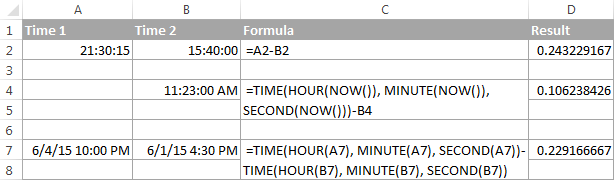The decimals in column D are perfectly true but not very meaningful. To make them more informative, you can apply custom time formatting with one of the following codes:

 Time code Explanation h Elapsed hours, display as 4. h:mm Elapsed hours and minutes, display as 4:10. h:mm:ss Elapsed hours, minutes and seconds, display as 4:10:20.

To apply the custom time format, click Ctrl + 1 to open the Format Cells dialog, select Custom from the Category list and type the time codes in the Type box. Please see Creating a custom time format in Excel for the detailed steps.

And now, let's see how our time difference formula and time codes work in real worksheets. With Start times residing in column A and End times in column B, you can copy the following formula in columns C though E:

`=\$B2-\$A2`

The elapsed time is displayed differently depending on the time format applied to the column: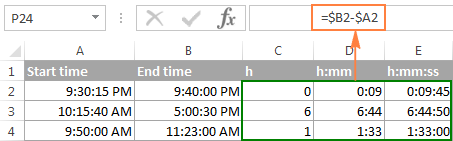Note. If the elapsed time is displayed as hash marks (#####), then either a cell with the formula is not wide enough to fit the time or the result of your time calculations is a negative value.

#### Formula 2. Calculating time difference with the TEXT function

Another simple technique to calculate the duration between two times in Excel is using the TEXT function:

• Calculate hours between two times:

`=TEXT(B2-A2, "h")`

• Return hours and minutes between 2 times:

`=TEXT(B2-A2, "h:mm")`

• Return hours, minutes and seconds between 2 times:

`=TEXT(B2-A2, "h:mm:ss")`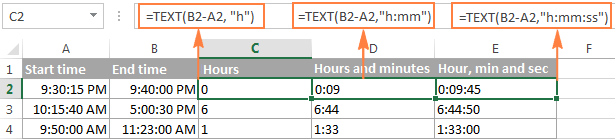Notes:

• The value returned by the TEXT function is always text. Please notice the left alignment of text values in columns C:E in the screenshot above. In certain scenarios, this might be a significant limitation because you won't be able to use the returned "text times" in other calculations.
• If the result is a negative number, the TEXT formula returns the #VALUE! error.

#### Formula 3. Count hours, minutes or seconds between two times

To get the time difference in a single time unit (hours ,minutes or seconds), you can perform the following calculations.

Calculate hours between two times:

To present the difference between two times as a decimal number, use this formula:

=(End time - Start time) * 24

Supposing that your start time is in A2 and end time in B2, you can use a simple equation B2-A2 to calculate the difference between two times, and then multiply it by 24, which is the number of hours in one day:

`=(B2-A2) * 24`

To get the number of complete hours, use the INT function to round the result down to the nearest integer:

`=INT((B2-A2) * 24)`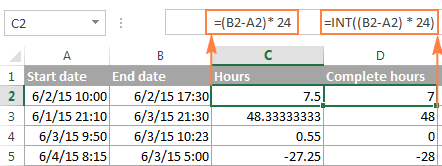Total minutes between two times:

To calculate the minutes between two times, multiply the time difference by 1440, which is the number of minutes in one day (24 hours * 60 minutes = 1440).

=(End time - Start time) * 1440

As demonstrated in the following screenshot, the formula can return both positive and negative values, the latter occur when the end time is less than the start time, like in row 5:

`=(B2-A2)*1440`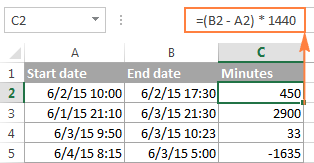Total seconds between times:

To get the total seconds between two times, you multiply the time difference by 86400, which is the number of seconds in one day (24 hours * 60 minutes * 60 seconds = 86400).

=(End time - Start time) * 86400

In our example, the formula is as follows:

`=(B2-A2)* 86400`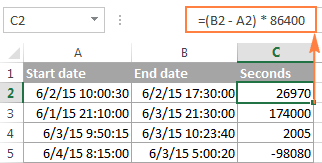Note. For the results to display correctly, the General format should be applied to the cells with your time difference formula.

#### Formula 4. Calculate difference in one time unit ignoring others

To find the difference between 2 times in a certain time unit, ignoring the others, use one of the following functions.

• Difference in hours, ignoring minutes and seconds:

`=HOUR(B2-A2)`

• Difference in minutes, ignoring hours and seconds:

`=MINUTE(B2-A2)`

• Difference in seconds, ignoring hours and minutes:

`=SECOND(B2-A2)`

When using Excel's HOUR, MINUTE and SECOND functions, please remember that the result cannot exceed 24 for hours and 60 for minutes and seconds.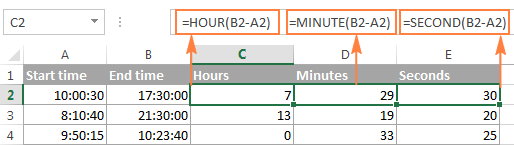Note. If the end time is less than the start time (i.e. the result of the formula is a negative number), the #NUM! error is returned.

#### Formula 5. Calculate elapsed time from a start time to now

In order to calculate how much time has elapsed since the start time to now, you simply use the NOW function to return today's date and the current time, and then subtract the start date and time from it.

Supposing that the beginning date and time is in call A2, the formula below returns the following results, provided you've applied an appropriate time format to column B, h:mm in this example:

`=NOW()-A2`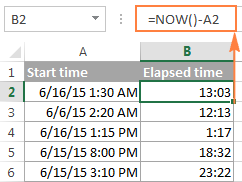In case the elapsed time exceeds 24 hours, use one of these time formats, for example d "days" h:mm:ss like in the following screenshot: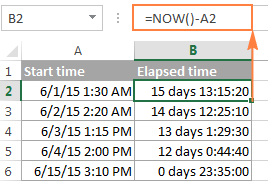If your starting points contain only time values without dates, you need to use the TIME function to calculate the elapsed time correctly. For example, the following formula returns the time elapsed since the time value in cell A2 up to now:

`=TIME(HOUR(NOW()), MINUTE(NOW()), SECOND(NOW())) - A2`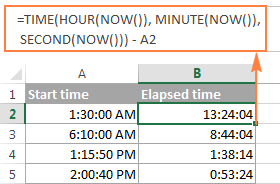Note. The elapsed time is not updated in real-time, it refreshes only when the workbook is reopened or recalculated. To force the formula to update, press either Shift + F9 to recalculate the active spreadsheet or hit F9 to recalculate all open workbooks.

#### Formula 5. Display time difference as "XX days, XX hours, XX minutes and XX seconds"

This is probably the most user-friendly formula to calculate time difference in Excel. You use the HOUR, MINUTE and SECOND functions to return corresponding time units and the INT function to compute the difference in days. And then, you concatenate all these functions in a single formula along with the text labels:

`=INT(B2-A2) & " days, " & HOUR(B2-A2) & " hours, " & MINUTE(B2-A2) & " minutes and " & SECOND(B2-A2) & " seconds"`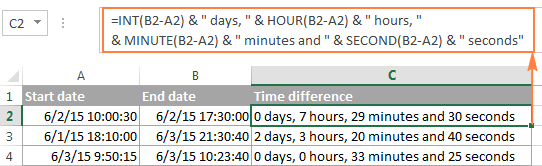To instruct your Excel time difference formula to hide zero values, embed four IF functions into it:

`=IF(INT(B2-A2)>0, INT(B2-A2) & " days, ","") & IF(HOUR(B2-A2)>0, HOUR(B2-A2) & " hours, ","") & IF(MINUTE(B2-A2)>0, MINUTE(B2-A2) & " minutes and ","") & IF(SECOND(B2-A2)>0, SECOND(B2-A2) & " seconds","")`

The syntax may seem excessively complicated, but it works :)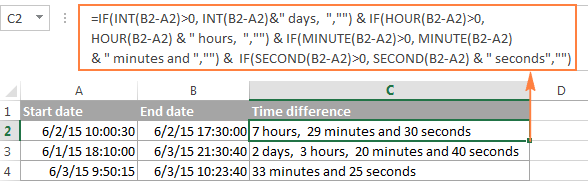Alternatively, you can calculate time difference by simply subtracting the start time from the end time (e.g. `=B2-A2`), and then apply the following time format to the cell:

d "days," h "hours," m "minutes and" s "seconds"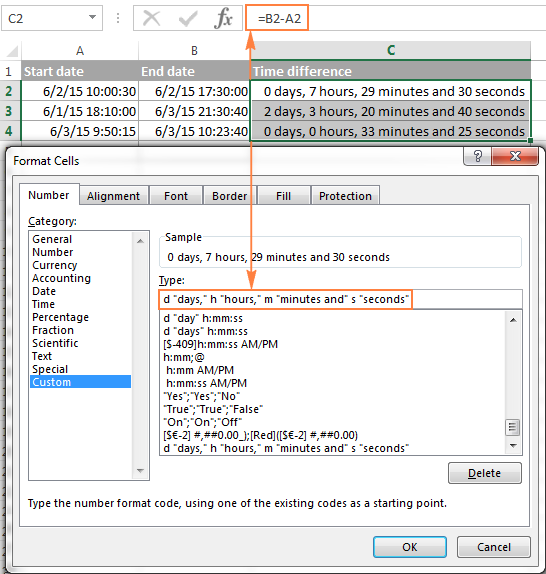An advantage of this approach is that your result would be a normal time value that you could use in other time calculations, while the result of the complex formula discussed above is a text value. A drawback is that the custom time format cannot distinguish between zero and non-zero values and ignore the latter. To display the result in other formats, please see How to show time over 24 hours, 60 minutes, 60 seconds.

## How to calculate and display negative times in Excel

When calculating the time difference in Excel, you may sometimes get the result as ###### error because the difference is a negative time. But is there a way to show negative times properly in Excel? Of course, there is a way, and even more than one :)

#### Method 1. Change Excel Date System to 1904 date system

The fastest and easiest way to display negative time normally (with a minus sign) is switching to the 1904 date system. To do this, click File > Options > Advanced, scroll down to the When calculating this workbook section and put a tick in the Use 1904 date system box.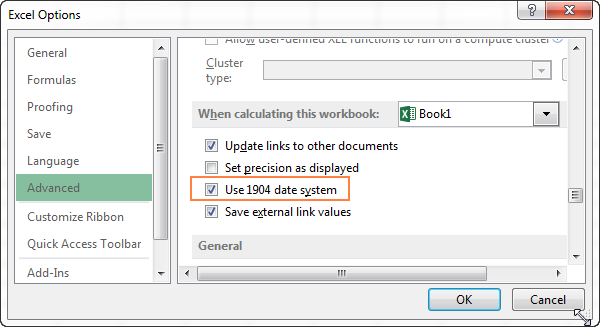Click OK to save the new settings, and from now on negative times will be displayed correctly, like negative numbers: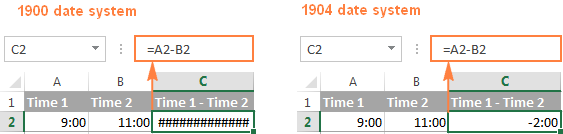#### Method 2. Calculate negative time in Excel with formulas

Is changing Excel's default Date System is not an option, then you can force negative times to display properly using one of the following formulas:

`=IF(A2-B2>0, A2-B2, "-" & TEXT(ABS(A2-B2),"h:mm"))`

`=IF(A2-B2>0, A2-B2, TEXT(ABS(A2-B2),"-h:mm"))`

Both formulas check if the time difference (A2-B2) is greater than 0, and if it is they return that difference. If the time difference is less than zero, the first formula calculates the absolute difference and concatenates the minus sign. The second formula yields exactly the same result by using a negative time format "-h::mm".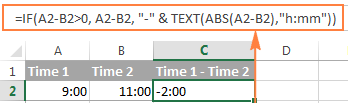Note. Please keep in mind that unlike the first method that treats negative times as negative numeric values, the result of the TEXT function is always a text string that cannot be used in calculations or other formulas.

## Adding and subtracting time in Excel

Basically, there are 2 ways to add and subtract time in Excel:

• Using the TIME function
• Using arithmetic calculations based on the number of hours (24), minutes (1440) and seconds (86400) in one day

The `TIME(hour, minute, second)` function makes Excel time calculations really easy, however it does not allow adding or subtracting more than 23 hours, or 59 minutes, or 59 seconds. If you are working with bigger time intervals, then use one of the arithmetic calculations demonstrated below.

### How to add or subtract hours to time in Excel

To add hours to a given time in Excel, you can use one the following formulas.

TIME function to add under 24 hours

=Start time + TIME(N hours, 0, 0)

For example, if your start time is in cell A2, and you want to add 2 hours to it, the formula is as follows:

`=A2 + TIME(2, 0, 0)`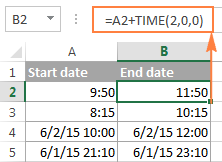Note. If you try adding more than 23 hours with the TIME function, the specified hours will be divided by 24 and the remainder will be added to the start time value. For example, if you try to add 25 hours to "6/2/2015 10:00 AM" (cell A4) using the formula `=A4 + TIME(25, 0, 0)`, the result will be "06/02/2015 11:00", i.e. A4 + 1 hour.

Formula to add any number of hours (under or over 24 hours)

The following formula has no limitations to the number of hours you want to add:

= Start time + (N hours / 24)

For example, to add 28 hours to the start time in cell A2, enter the following formula:

`=A2 + (28/24)`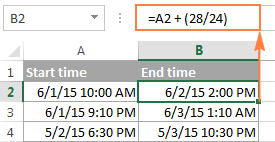To subtract hours from a given time, you use analogous formulas, and just replace "+" with the minus sign:

For example, to subtract 3 hours from the time in cell A2, either of the following formulas will do:

`=A2-(3/24)`

`=A2-TIME(3,0,0)`

To subtract more than 23 hours, use the first one.

### How to add / subtract minutes to time in Excel

To add minutes to a given time, employ the same techniques that we've just used for adding hours.

To add or subtract under 60 minutes

Use the TIME function and supply the minutes you want to add or subtract in the second argument:

=Start time + TIME(0, N minutes, 0)

And here are a couple of real-life formulas to calculate minutes in Excel:

To add 20 minutes to the time in A2: =A2 + TIME(0,20,0)

To subtract 30 minutes from the time in A2: =A2 - TIME(0,30,0)

To add or subtract over 60 minutes

In your calculation, divide the number of minutes by 1440, which is the number of minutes in a day, and add the quotient to the start time:

=Start time + (N minutes / 1440)

To subtract minutes from time, simply replace plus with the minus sign. For example:

To add 200 minutes: `=A2 + (200/1440)`

To subtract 300 minutes: `=A2 -(300/1440)`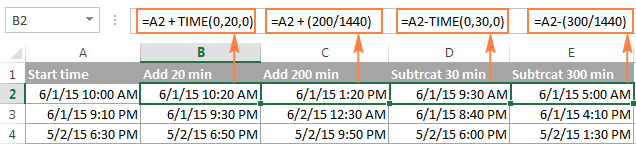### How to add / subtract seconds to a given time

Second calculations in Excel are done in a similar fashion.

To add under 60 seconds to a given time, you can use the TIME function:

=Start time + TIME(0, 0, N seconds)

To add more than 59 seconds, use the following formula:

=Start time + (N seconds / 86400)

To subtract seconds, utilize the same formulas with the minus sign (-) instead of plus (+).

In your Excel worksheets, the formulas may look similar to these:

To add 30 seconds to A2: `=A2 + TIME(0,0,31)`

To add 1200 seconds to A2: `=A2 + (1200/86400)`

To subtract 40 seconds from A2: `=A2 - TIME(0,0,40)`

To subtract 900 seconds from A2: `=A2 - (900/86400)`

## How to sum time in Excel

The Excel sum time formula is the usual SUM function, and applying the proper time format to the result is what does the trick.

Supposing you have a few project times in column B and you want to add them up. You write a simple SUM formula like the one below and get the result in the default format such as hh:mm:ss.

`=SUM(B2:B4)`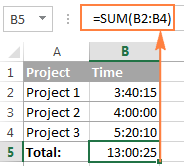In some cases the default time format works just fine, but sometimes you may want more, for example to display the total time as minutes and seconds, or seconds only. The good news is that no other calculations are required, all you have to do is apply custom time format to the cell with the SUM formula.

Right click the cell and select Format Cells in the context menu, or press Ctrl + 1 to open the Format Cells dialog box. Select Custom from the Category list and type one of the following time formats in the Type box:

• To display total time as minutes and seconds: [m]:ss
• To display total time as seconds: [ss]

The result will look as follows: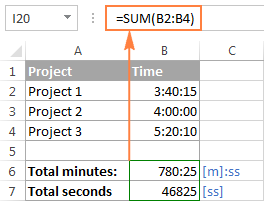### How to sum over 24 hours in Excel

In order to add up more than 24 hours, you use the same SUM formula as discussed above, and apply one of the following time formats to the cell:

 Format Displays as Explanation [h]:mm 30:10 Hours and minutes [h]:mm:ss 30:10:20 Hours, minutes and seconds [h] "hours", mm "minutes", ss "seconds" 30 hours, 10 minutes, 20 seconds d h:mm:ss 1 06:10:20 Days, hours, minutes and seconds d "day" h:mm:ss 1 day 06:10:20 d "day," h "hours," m "minutes and" s "seconds" 1 day, 6 hours, 10 minutes and 20 seconds

To see how these custom time formats may look like in your Excel worksheet, please have a look at the screenshot below, where the same SUM formula is entered in cells A9 to A13:

`=SUM(\$B\$2:\$B\$4)`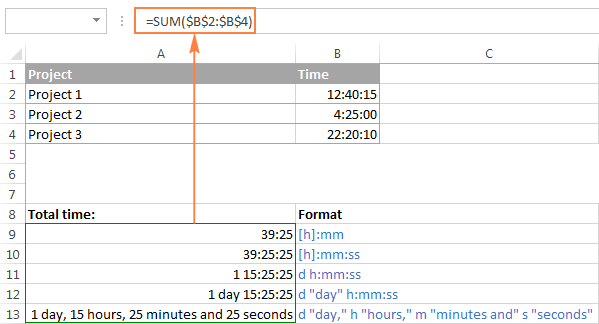Note. The above custom time formats work for positive values only. If the result of your time calculations is a negative number, e.g. when you are subtracting a bigger time from a smaller time, the result will be displayed as #####. To display negative times differently, please see custom format for negative time values.

Also, please keep in mind that the time format applied to a cell changes only the display presentation without changing the cell's value. For example, in the screenshot above, cell A13 looks like text, but in fact it's a usual time value, which is stored as a decimal in the internal Excel system. Meaning, you are free to refer to that cell in other formulas and calculations.

For more information, please see How to calculate and show over 24 hours, 60 minutes, 60 seconds.

## Date & Time Formula Wizard - quick way to calculate times in Excel

Now that you know a bunch of different formulas to add and subtract times in Excel, let me show you the tool that can do it all. Okay, almost all :)

Here comes Ablebit's Date & Time Formula Wizard for Excel:In the Date & Time Wizard dialog window, you switch to the Add or Subtract tab, depending on which operation you want to perform, and do the following:

• Click the Show time fields link in the left part of the window.
• Supply values or cell references for the formula arguments. As you fill in the argument boxes, the wizard builds the formula in the selected cell.
• When finished, click the Insert Formula

That's it! For example, this is how you can add the specified number of hours, minutes and seconds to the time in A4: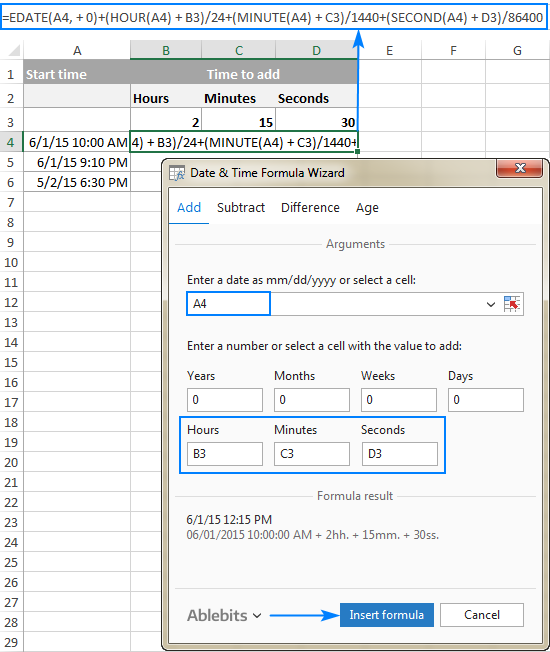If you need to copy the formula to other cells, fix all references except the cell containing the original time (A4) with the \$ sign like shown in the screenshot below (by default, the wizard always uses relative references). Then double-click the fill handle to copy the formula down the column and you are good to go!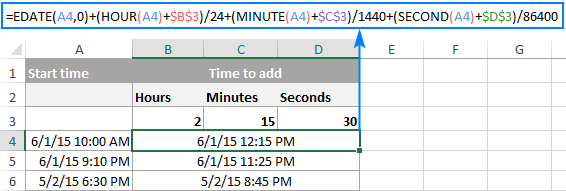Besides time calculations, the wizard can also add and subtract dates, get the difference between two dates, and calculate age from the birthdate.

If you are curious to try this tool in your own worksheets, you are welcome to download the evaluation version of our Ultimate Suite below.

This is how you calculate time in Excel worksheets. To learn other ways to manipulate dates and times in Excel, I encourage you to check out the resources at the end of this article. I thank you for reading and hope to see you on our blog next week!

Excel Time Calculations - formula examples (.xlsx file)
Ultimate Suite - trial version (.exe file)

### Excel Date and Time tutorials:

1. Hi,

I'm trying to create a time sheet to be able to track my breaks, also at work I have to track the total minutes that I'm in different meetings throughout the day. For example I have track the total minutes I'm on a phone call, total minutes I'm in different meetings, etc. (in total there could be up to 16 total different things I have to keep track of throughout my shift), also want to track total hours worked for the day and the week and any over time that is being done. For example this is an example of a break down of what I have to keep track of and what my work day can look like and how I have to track it,

Clock In: 6a
Office Time 1: 6am- 7:45am
Break 1: 7:45am-8am
Phone Time 1: 8am-9am
Break 2: 9am-9:05a
Phone Time 2: 9:05a-10a
Break 3: 10a-10:05a
Phone Time 3: 10:05a-11:15a
Meeting 1: 11:15a-11:30a
Lunch (Deducting 30 min. for Lunch) : 11:30a-12p
Meeting 2: 12p-12:15p
Phone Time 4: 12:15p- 1p
Break 4: 1p-1:15p
Phone Time 5: 1:15p-2p
Meeting 3: 2p-2:15p
Clock Out: 2:30p
Overtime (Phone Time 5): 2:30p-4p

The time of the meetings, breaks and phone time may vary and aren't always consistent. I hope I provided an example with enough detail to help create a formula for this. Thank you in advance!

• Hi! You write the time intervals as text. Therefore, it is not possible to find the sum of the times from your data. If the start time and end time are written in different cells, you can find the duration of each action as described in the article above. You can then use the SUMIF function to calculate, for example, the sum of the "Break" time. I hope I answered your question. If something is still unclear, please feel free to ask.

2. Hi, I am looking for a formula to calculate hours worked (end time-start time), but also to subtract 30 minutes if cell B isn't blank. So basically if there is a value in cell B, then subtract 30 minutes from the total time worked. Otherwise, if cell B is blank then don't subtract 30 mins.

• I think I figured it out:
=IF(ISBLANK(B2), C2-A2, C2-A2-TIME(0, 30, 0))

3. Hello, I have a problem I cannot wrap my head around. I need to calculate overtime (time worked over 8 hours) that happened at night (between 22:00 and 6:00) and separately overtime that happened during the day.
I have start of the work (I6), end of the work (J6), total time worked (K6), total overtime (L6) and how much time I worked during the night (O6).
I want to calculate specifically how many overtime hours happened during the night and how many happened during the day.
Thanks for any help.

• Hi! If I understand your task correctly, try the following formulas:
Day overtime:
=IF(M6>0,IF(L6>M6,L6-M6,0),L6)
Night overtime:
=L6-O6

• Hey! Thanks a lot for an answer. As much as I like simplicity of this solution (and I got really excited to see it working), it doesn't work for all the cases. For example starting work at 9PM and working until 9AM shows 4 night OT hours, but it should be 1 night OT hour and 3 day OT hours.

• For those interested: Formulas that ended up working for me in all the cases were:
Day overtime (M6);
=IF(I6<=J6,MIN(L6,MAX(0,"22:00"-I6-"8:00")),MIN(L6,MAX(0,J6-"06:00")+MAX("22:00"-(I6+"8:00"),0)))
Night overtime(N6):
=L6-M6
And no, I didn't think of that myself x)

4. I need help regarding my formula.
cell B3: 1/5/2023 18:38
cell C3: 1/5/2023 18:47
cell D3: 0 days, 0 hours, 9 minutes, and then the result for cell E3: Failed,
but the formula for cell E3 is =IFERROR(IF(D3<="0 days 0 hours 15 mins","Passed","Failed"),""
formula for D3 is =IFERROR(INT(C3-B3)& "days" & HOUR(C3-B3)& "hrs" & MINUTE(C3-B3)& "mins","")
The format for cells B3 and C3 is custom "mm/dd/yyyy hh:mm."
should be the correct result for cell E3 is passed if the formula is less than 15 minutes.

• Hi! There is a text in cell D3. You cannot do any mathematical operations on the text, and you cannot do comparisons < and >. Try this formula:

=IFERROR(IF(C3-B3<=TIME(0,15,0),"Passed","Failed"),"")

5. HI

I am looking to complete the following in time value

start time
finish time
total hours (finish time less start time)

I need then if the total hours are greater than 0, deduct 30 minutes lunch

I cannot figure out the latter part :(

• Hi! Please re-check the article above since it covers your case. To subtract 30 minutes, use the TIME function:

=IF(B1>A1,B1-A1-TIME(0,30,0),"")

6. Hi
So let's just say I have data in C:C
All data is represented as h:m:ss AM/PM based on a form
eg. 1:58 AM, 3:20PM, 2:23PM, 2:39PM.... the list goes on
How do I count the hour and any minute associated during the AM/PM

eg. (1) 1AM
(1) 3PM
(2) 2PM

I have used =COUNTIF(C:C,"="&TIME(12,0,0))
to check the total pm

However I was experimenting with those formulas and I've gotten close to it but when proof checking it's not giving me the accurate data per hours

Please feel free to help/ correct me

7. I work 10 hour shifts. Overtime start after 8:48. There are times I exceed my shift length or working less hours
Both formulas in H13 and H14 calculate correct, individually. Not vice versa
Have to combine both formulas Fig H13 and H14 to get one formula in H
Fig13. 10:00 hours shift minus 8:48 = 1:12 Actual overtime H13
Fig14. 10:00 hours shift, so 11:00 minus 8:48 = 2:12 Actual overtime H14

Figure H13
C13 D13 E13 F13 G13 H13
Shift length Start time End time Hour Work Overtime Actual overtime
10:00 06:00 15:00 09:00 00:12 01:12
H13 =IF(C13>F13-TIME(8,48,0)>=TIME(1,0,0),C13-TIME(8,48,0),0

Figure H14
C14 D14 E14 F14 G14 H14
Shift length Start time End time Hour Work Overtime Actual overtime
10:00 06:00 17:00 11:00 02:12 02:12
H14 =IF(C14>F14-TIME(8,48,0)>=TIME(1,0,0),F14-TIME(8,48,0),0)

• Hi! Sorry, it's not quite clear what you are trying to achieve. Explain the result you want if your two formulas use the same conditions.

• If i work 9 hours then the formula works in H13 shows the right time 1:12. Fig H13 Shift length - 8:48
So does the formula in H14. Hours worked - 8:48
But when i insert H13 formula in H14, i get 1:12 hours instead of 2:12.
Same happens if i insert H14 formula in H13, i get 00:12 hours instead of 1:12
So if i want the right time to show in H, i have to change the formula every time.
The last piece of the formula in C13-Time(8,48,0)0) Shift length - 8:48, i have to change to F14-Time(8,48,0)0) Hours worked - 8:48
In H13 i have to deduct 8:48 from Shift length
In H14 i have to deduct 8:48 from Hours worked
That's why i need one formula in H so i don't have to change the formula every time i work less or more than 10 hours
Hope my explanation helps

• I’m not sure I got you right, since the description you provided is not entirely clear. However, it seems to me that the formula below will work for you:

=IF(F13<=TIME(10,0,0), IF(C13>F13-TIME(8,48,0)>=TIME(1,0,0),C13-TIME(8,48,0),0), IF(C13>F13-TIME(8,48,0)>=TIME(1,0,0),F13-TIME(8,48,0),0))

Combine two formulas using the IF function to perform calculations on the condition. I hope I guessed this condition correctly.

• Thanks so much

Tried it and its working 100 percent for me.

• HOW TO CALCULATE STAR PUCNCHING TIME TO END PUNCHING TIME
Emp No date time "total
work hr"
123 14/9/23 10:00:10 AM
124 14/9/23 10:00:10 AM
123 14/9/23 13:00:10 PM
124 14/9/23 13:45:10 PM
123 14/9/23 18:08:45 PM

• I’m sorry, but your task is not entirely clear to me. Could you please describe it in more detail? What result do you want to get? Give an example of the expected result.

• Hi! need help on what formula to use to get the total overtime. our official time out is 8:00PM and our store closes at 9:00pm but when we exceed at 9:15PM it is considered as 1hr & 30 minutes overtime already. Can't figure out on how to set the formula that authomatically counts it as 1.5 hrs overtime.
Given F6 as start time and G6 as end time.

• Hi! If I understand the question correctly, use the IF function to compare the times and the TIME function to set the specific time value. For example:

=IF(G6>TIME(21,0,0),TIME(1,30,0),0)

8. Hello,

I want to find the STARTING CLOCK time for a sequence of tasks where I know and can enter the DURATION time of the needed tasks.

I do simple subtraction of durations from one column and Excel is correclty displaying the correct startging CLOCK time in another column, but the moment a duration puss the clock backwards past midnight it breaks and shows a line of #######

Any suggestions?

• Hi! If the end time is longer than the start time, then you need to add 1 day to their difference:

=IF(A1 > B1,B1-A1+1,B1-A1)

9. I'm trying to calculate the decimal hours and minutes worked when the shift starts at 10:00am an goes through to 2:00am the following morning
This is what I get:-
Open Hours
A B (B-A) =C
Open Close Hours ON /Day
10:00 2:00 -8.0 hrs

Is there a formulae that will show the correct decimal hrs:mm worked when spanning 2 days. (I am not concerned about the days) and jusst enter the hours and sec using as time filter eg 10:30
Open Hours
A B C=?
Open Close Hours ON /Day
10:00 2:00 16.0 hrs

10. Hello

I am working on a timesheet for staff and I am looking for a way to calculate how much time is spent working after 8pm if someone works that long. It would be in hh:mm.

• Hi! If I understand your task correctly, try the following formula:

=IF(A1>TIME(20,0,0),A1-TIME(20,0,0),0)

Use the TIME function to set 8PM, and the IF function to find the difference if the end time of A1 is greater than 8PM.

11. I am trying to use Excel 2007 to identify and display fastest times for each stroke, distance, and course (yards/meters) from a sheet with multiple meets, each with multiple strokes and distances, courses and times (formatted in min:seconds:hundredths (mm:ss:00)). I hoped to use Min(IF with an array function but can' get it to work. The function looks like this: (in a different version I used = instead of commas)

{=MIN(IF(D4:D100,D4,IF(E4:E100,E4,IF(F4:F100, F7,IF(F4:F100,F4,G4:G100)))))}

Where D = stroke (Free, Back, Breast, etc.) , E = Event distance (50, 100, 200, etc., F= course (yards or meters), and G = times achieved in minutes,seconds, and hundredths or seconds and hundredths where applicable.

The result I keep getting is 0:00.00 instead of the fastest time.

I'll have separate functions for each combination of stroke, distance, course. Ideally, I would like to grab the data from a separate sheet where I enter meet results but that is the least of my problems

• Hi! If I understand your task correctly, the following formula should work for you:

=MIN(IF(((\$D\$4:\$D\$10=D4)*(\$E\$4:\$E\$10=E4)*(\$F\$4:\$F\$10=F4)*G4:G10)>0, ((\$D\$4:\$D\$10=D4)*(\$E\$4:\$E\$10=E4)*(\$F\$4:\$F\$10=F4)*G4:G10), ""))

In your Excel, you need to enter this formula as an array formula.

• Thanks!

I haven't tried your version yet to see how it differs, but think by using TIMEVALUE I was able to omit the the first expression. I stumbled on my version after hours searching the web and experimenting before I saw yours. (As you can see, my data is on a separate sheet named Log.)

{=MIN(IF(Log!\$D\$4:\$D\$39=Log!\$D\$5,IF(Log!\$E\$4:\$E\$39=Log!\$E\$7,IF(Log!\$F\$4:\$F\$39=Log!\$F\$4,TIMEVALUE(Log!\$G\$4:\$G\$39)))))}

My next problem is how to automatically retrieve the date of the fastest time so it displays on my summary sheet . I entered dates into the Log as M/DD/YYYY and formatted to display as dd:MMM:YYYY. I used a single leading quotation mark to prevent the time from changing to a date when I click on it and to suppress the leading zero.

I tried using a modified version of the min. time function, but that returned the oldest (smallest) date in the range : ~ ) then I tried using a couple of simple IF formulas: IF( G - B = 0) , B = G, etc. they all returned either "False" or "Value" I think my problem is with TIME formatting

• Hi! Single leading quotation mark turns the date into text.

12. My job keeps a running total of time hours and minutes. For example my running total 2 months ago was 1410:51, last month my total was 1486:33. How do I find the difference between the 2 without excel converting it to days?

• You will want to set the number format to the following: [h]:mm which will show as 75:42

If you leave out the square brackets around the H, then it will show the time as an hour on a clock instead of the total hours. That will show as 3:42.

To change the format go to Home > Number then click the little arrow in the bottom right. In the left box click "Custom" then type in your custom number format.

13. I am trying to calculate my time throughout the day which includes a clocked in and out break. But with my formula it is showing what should just be an 8.30 day worked as 8.50. How do I fix this?

• How can I guess what formula and data you are using?

14. Hi Alexander Trifuntov/Ablebits Team.
I Currently have a column with the data of time elapsed for cars parking in a parking lot. format 00:00:00 H:M:S
i created the column using this formula =text(P665-L665-int(P665-L665),"HH:MM:SS")
and then formatted the column with the basic format function into Format>Number>Duration

i am trying to count the frequency that the time elapsed is over 2 hours (02:00:00). meaning the number of times a vehicle stayed longer than 2 hours.

any assistance would eb deeply appreciated

• Hi! To count values by condition, use the COUNTIF function. For your case, if the time is more than 2 hours:

=COUNTIF(A2:A20,">="&TIME(2,0,0))

15. hello,

I am looking for a cycle time formula for seconds. I work in manufacturing and have to track cycle times between pieces produced and then separately track the average time spent per piece.

• Hi! Have you tried the ways described in this blog post? If they don’t work for you, then please describe your task in detail, I’ll try to suggest a solution.

16. Hello,

I want to be able to create "out" times on a work schedule. Basically any time in a 12hour format input for the "in" column would auto populate an "out" time by adding 6 hours to the input.

Thank you

• Hi! Pay attention to the following paragraph of the article above: How to add or subtract hours to time in Excel.

17. Hi! I am needing to calculate an arrival time for a surgical unit. We use the Time of surgery (in 24hr time) + duration of surgery (in minutes) + 1hour for recovery. I'd like to program this calculation into a spreadsheet that we can use for patient flow. Ex. surgery time 0830, duration of 120 minutes + 60 minutes of recovery = arrival time of noon (1130). How can I write a formula to make these conversions properly?
Thank you!
Karin

• Hi!
If I understand your task correctly, the following formula should work for you:

=A1+(120+60)/1440

A1 - surgery time

18. I am working with a time sheet. I want a formula. Our working hours is 9:00 hrs. So, if a person works for 9:00hrs or more, I want to map it to 8:00 hrs and remaining in overtime cell. can you please help?

• If you want a formula, explain in detail what result you want. The information you provided is not enough to understand your case and give you any advice.

• The Formula I want is to map 9.00hrs or more to 8 hrs.

• To compare two time values and do some action depending on the result, use the IF function.

19. I am trying to figure out a formula for our time sheets. We have the work hours, that isn't the issue. My problem is converting the work hours to clock hours. For example someone works 7:30 hours, that is how we get it I have to then convert it to 7.50 to figure payroll. Does that make since what I am asking. Example of what we do
5/15/2023 1:15 PM 3:14 PM 1:59 1.98
Date clock in clock out hours worked I convert this manually looking for a way to automatically do this
Thank you

20. Hello, I am trying to subtract overtime hours from regular hours that are on 2 different cells but I keep getting #VALUE error

Example: In cell A2 I have :15 for total worked hours and in cell B2 I have :00 for regular work hours
The difference would be =A2-B2 to get a total of :15, meaning 2 hours and 15 mins of overtime but I keep getting #value error.

note: I am not working with date or time formats I would say, since they are hours worked, not time of the day

21. Hi Alexander Trifuntov/Ablebits Team.
With the condition:
The working hours are Monday to Saturday from 09:00 AM - 06:00 PM and Sunday from 02:00 PM - 08:00 PM.
Holiday only on public holiday (e.g. May 1, December 25)
Ticket received on April 30, 2023 at 05:30 PM
Ticket responded on May 2, 2023 at 09:30 AM

Could you please help me, what is the Excel formula to calculate the timestamp difference above (in hours) excluding public holidays?

• Hi! Your request goes beyond the advice we provide on this blog. This is a complex solution that cannot be found with a single formula.

• Hi Alexander, really appreciate the response.
How about with more simple condition:

The working hours from Monday to Sunday from 09:00 - 18:00
Full holiday (no working) only on public holiday such as January 1, May 1, December 25
A. Ticket received on Sunday, April 30, 2023 at 17:30 B. Ticket responded on Tuesday, May 2, 2023 at 09:30

What is the Google sheets formula to calculate the working hours of timestamp difference between ticket received and ticket responded above (in minutes)?

The expected answer is 60 minutes: I have 30 minutes left on Sunday but still not responded, skip on Monday because it is holiday, and used 30 minutes on Tuesday to reply it.

Tried to use NETWORKDAYS.INTL but it does not work. Really appreciate with your help.

22. Hi,
Can any one help me find solution for below

When I'm downloading attendance from biometric machine, I'm noticed that punch in punch out will showing in single cell, now I want to find a formula for generate total working hour and OT,
The main problem is I need to check each cell for total 30 days in month for a single person,
And we have more then 400 people working, I'm calculating manually total 30x400, may i know is their any alternate solution
Ex: 6:00 17:37

I'm get like this

• Unfortunately, this information is not enough to understand what you need. Please describe your problem in more detail. Include an example of the source data and the result you want to get.

• Sir, there is no option for uploading an example pic, can you please suggest me alternate way

23. I'm trying to calculate running hours for a fleet of vehicles over a week, however, I am encountering a problem - All vehicles are on the same sheet, each recognized by a unique code (multiple pieces of data are recorded for the vehicles each day, based on which vehicles are used. Not all vehicles are run every day.) I'm trying to implement a formula that can be carried from one week to the next and doesn't need to be re-coded each week based on the location of the vehicles on the sheet.

In a nutshell, I need a variant on the "CountIf" or 'SumIf" formulas so that the program can recognize the vehicle codes, and then calculate the difference between the smallest and greatest number of hours. Would this be possible?

• Hi! I don't have your data, so I can't recommend any formula. But here is a detailed instruction for the SUMIF function to calculate the amount of time for each vehicle. If this is not what you need, explain in more detail.

24. Hi, my organisation requires we complete our time sheets using excel. The date is selected via a drop down menu where it then automatically populates the next field with the actual day. My big problem are the start and end times where the columns AM and PM columns are separated and once again are selected by a drop list where either AM or PM can be selected. We are paid from start to end time over that 24 hour day minus the number of hours we actually slept during that overnight shift, In the next column after sleep time, I want to input the required formula to provide the number of hours accumulated as awake, (start to End on the following day) minus the sleep time. How would this be done noting I can't change the existing spreadsheet layout and only add the additional column after sleep time with my own calculations. Thanks

Date SHIFT Client Start End Shift Type Sleep Time
12/04/2023 Wednesday ACA Bob 10.45 AM 9.00 AM Day Shift 11pm to 6am
13/04/2023 Thursday ACA Bob 9.00 AM 9.00 AM Sleepover 11.45pm to 6am
14/04/2023 Friday ACA Bob 9.00 AM 9.00 AM Sleepover 11pm to 6am

• Hi! You can calculate the number of hours like this:
(11pm - 10.45 AM) + (9.00 AM - 6am)
I can't give you the formula because I don't know what cells your data are written in.
If this does not help, explain the problem in detail.

25. Hello,

so I am trying to make a timesheet for my employee. I have their start time and end time of work. when I try to calculate total time they worked using timevalue formula, it gives me result in time format.

for example, I have start time on c2 which is 5:45am and end time on d2 which is 7:45am , so I am trying to calculate hrs he worked using formula, but end result is 2:00am.

how can I just get a hrs in Number but not in a time format.

• Hello!
If the end time is greater than the start time, then Excel cannot determine that the end time is already the next day. To correctly calculate the time difference in your case, use the date and time. You can also find useful information in this article: How to show over 24 hours, 60 minutes, 60 seconds in Excel.

26. Hi,

I am working on a document for my work to calculate times. Basically i need to have each block typeable for later use to where the calculation is still there for future use. That's the first hurdle. The second i have kind of figured out is to calculate the hours for next to be picked up (i.e. 4 hours , 5 hrs and so on. Then if the time falls into certain hours time frame i need it to factor the added time plus some because we cannot deliver at those times. Thank you

• Hi!
To understand what you want to do, give an example of the source data and the desired result.

27. Hello alexander,
I'm trying to calculate the duration for multiple tasks between differentes dates, like 04/04/2023 08:00:00 and 05/04/2023 10:00:00 in date format.
But i need to inlude only the working days and hours, which are:
Opening hours: 08:00 to 16:30
With mutiple breaks: 10:00 to 10:15 / 11:30 to 13:00 / 15:00 to 15:15.
The tasks varies wildy in lenght, it can range from 2 hours to 2-3 days.

Do you know a formula for this calcul?
Thank you

28. I'm trying to sum a total of each individual who has worked for the entire week. I have a data that I have calculated the daily working hours but I am unable to get a total of the entire week.

29. I'm trying to calculate the amount of time I worked down to the exact minute for the entire month, but when I try to sum it it just adds time to each other in a 24 hour format, starting me from 0 again after every 24 hours

30. Good Information regarding Duaration Calculation in all possible ways
Thanking You
Tandeep
St Joseph's School
Vijapur, Gujarat, India

31. Hello, I have huge time data in the format, 00:07:15:20. I want to perform sums, divide them by other number variables to find variable per time, but whenever I change the format it doesn't seem to work instead, it reverts back to a format like this "08/01/1900 08:15:15"

How do I resolve this?

• Hi!
Your task is not completely clear to me. Explain what your format means and what actions you want to perform. Give an example expected result.

32. Is there a way to set up a formula to calculate the elapsed hours/minutes only during certain timeframes/days?

I.e. We want to calculate elapsed time from order received to order sent out. But we only want the elapsed time to calculate from Monday - Friday 8am-4pm. So if an order comes in at 7pm on Monday night, we want the elapsed time to start calculating as of 8am on Tuesday and continuing to track business hours only until the order is sent out.

33. HOW TO TOTAL NUMBER OF HOURS AND MINUTES

• Have you tried the ways described in this blog post? If they don’t work for you, then please describe your task in detail.

34. Drives me nuts. I got time formatted in HH:mm:ss and I try to get the difference between two times in seconds. I always get the output mm:ss if I multiply it by 86400 I get zero.

Another problem I've encountered is that =(B2-((-9,59)/(465)*125)) outputs a value error. I don't get any of this.

• Hello!
Question 1: Try changing the cell format to General
Question 2: Check the value in cell B2. Check the decimal separator. Probably correct 9.59 You don't need so many brackets. =B2-(-9.59/465*125)

35. I am looking to scan a barcode in column A, that then produces the date and time it was scanned in column B. When I use the the formula =IF(A1="","",now()) in column B, and I scan a barcode into A1, the correct time comes up. now when the formula is copied down the column, B2 appears blank (as it should). Then when you scan a new barcode into A2 any amount of time later after the first time, the current time appears in B2 and B1 changes to the new current time.

I need a formula to calculate the time of the scan in the adjacent cell, that doesn't change unless the adjacent cell changes.

• Hi!
You can use a VBA macro to replace the formula with the current time when you enter a value in another cell.

• Hi
I’m trying to create a roster that calculates hours worked and automatically subtracts non paid breaks
For example if someone works 12pm-7pm it would be 7 hour in total but minus 1 hour for unpaid breaks so the excel sheet should show 6 hours instead of 7.
I can get the formula to add the hours but not sure what formulas to use to minus unpaid breaks

• Hi! Subtract 1 hour from the time difference. For example, by using the TIME function:

=End time - Start time - TIME(1,0,0)
or
=End time - Start time - 1/24